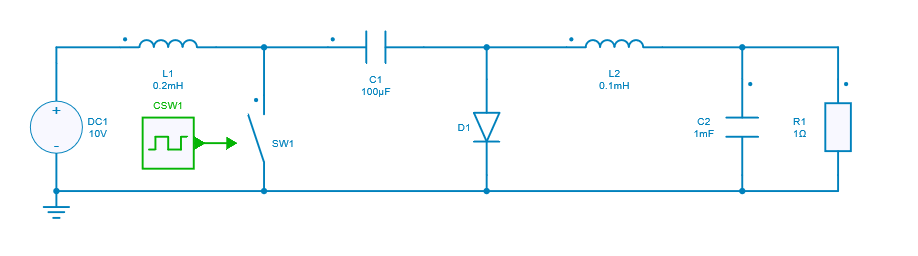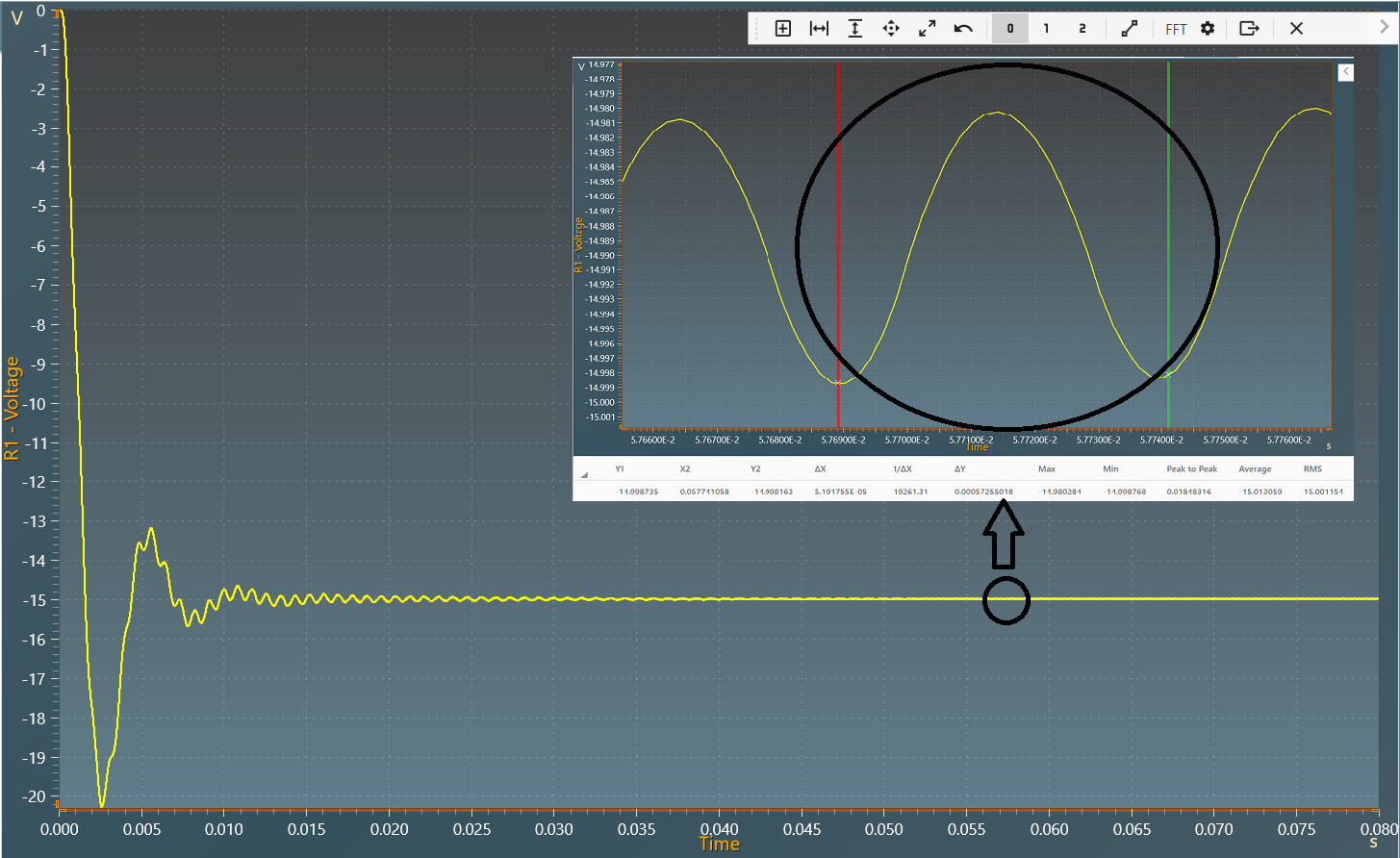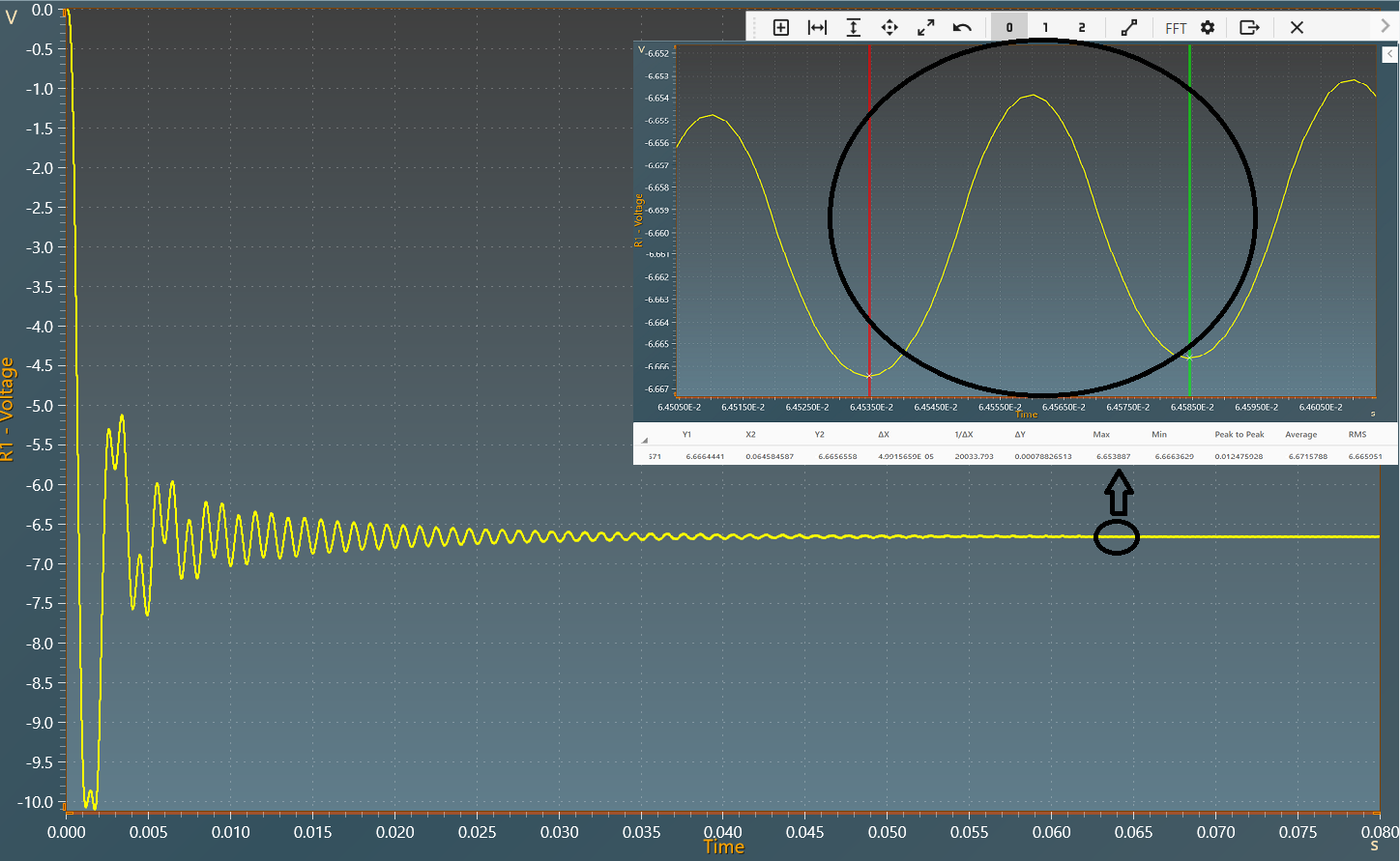Resources

# DC-DC Cuk converter

This example shows a DC-DC Cuk converter with:

• an input voltage of 10 V,
• output voltage of 15 V for duty cycle D = 0.6,
• a power of 225 W transferred ( $$P_{output}=P_{input}$$ ).

The non-isolated Cuk converter is a DC-DC power converter that, like a buck-boost converter, can produce an output voltage magnitude that is either greater or less than the input voltage magnitude. However, it is an inverter converter, so the output voltage is of opposite polarity with respect to the input voltage.

## Model

In this model, all components are ideal.When the switch is ON (closed), inductor L1 is directly connected to the input source. Therefore, the voltage across inductor is equal to the source voltage. Also, inductor L2 gets connected in series with capacitor C1 and C2 making the voltage across it is equal to the sum of output voltage and the voltage across C1.

However, when the switch is OFF (open), the inductor L1 is connected in series with input voltage and capacitor C1. In this case, the diode is forward biased and inductor L2 is directly connected to the output capacitor C2. In conclusion, the energy is transferred from inductor, capacitor C1 and the input dc source to the load when the switch is off.

## Switch control

The switch model can be directly connected with a control signal. For this control, we use a PWM block with an amplitude of 1, a duty cycle of 0.6 and 0.4 respectively, and a frequency of 20KHz.

Here are the values of the other components :

• Inductor L1: 0.2 mH,
• Capacitor C1: 100 µF,
• Inductor L2: 0.1 mH,
• Capacitor C2: 1mF,
• Load R1: 1 $$\Omega$$.

## Simulation

The results below show the output voltage across R1 with a duty cycle= 0.6. A zoom shows a measure of the average value of this voltage : -15 V.The results below show the output voltage across R1 with a duty cycle= 0.4. A zoom shows a measure of the average value of this voltage : -6.67 V.The results follow the equation: $$V_{out} = - {V_{in}}\times\frac{D}{1-D}$$

With:

• D: duty cycle
• $$V_{in}$$: Input voltage
• $$V_{out}$$: Output voltage